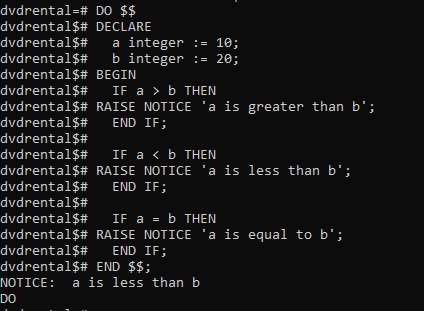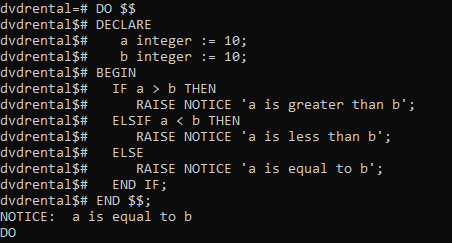Open in App
Not now

## Related Articles

• Write an Interview Experience

# PostgreSQL – IF Statement

• Last Updated : 22 Feb, 2022

PostgreSQL has an IF statement executes `statements` if a condition is true. If the condition evaluates to false, the control is passed to the next statement after the END IF part.

```Syntax:
IF condition THEN
statements;
END IF;
```

The above conditional statement is a boolean expression that evaluates to either true or false.

Example 1:

In this example, we declare two variables a and b. In the body of the block, we compare the value of a and b using the comparison operator >, < and = in the boolean expressions of the IF statements.

```DO \$\$
DECLARE
a integer := 10;
b integer := 20;
BEGIN
IF a > b THEN
RAISE NOTICE 'a is greater than b';
END IF;

IF a < b THEN
RAISE NOTICE 'a is less than b';
END IF;

IF a = b THEN
RAISE NOTICE 'a is equal to b';
END IF;
END \$\$;
```

Output:Example 2:

```DO \$\$
DECLARE
a integer := 10;
b integer := 10;
BEGIN
IF a > b THEN
RAISE NOTICE 'a is greater than b';
ELSIF a < b THEN
RAISE NOTICE 'a is less than b';
ELSE
RAISE NOTICE 'a is equal to b';
END IF;
END \$\$;
```

Output:My Personal Notes arrow_drop_up